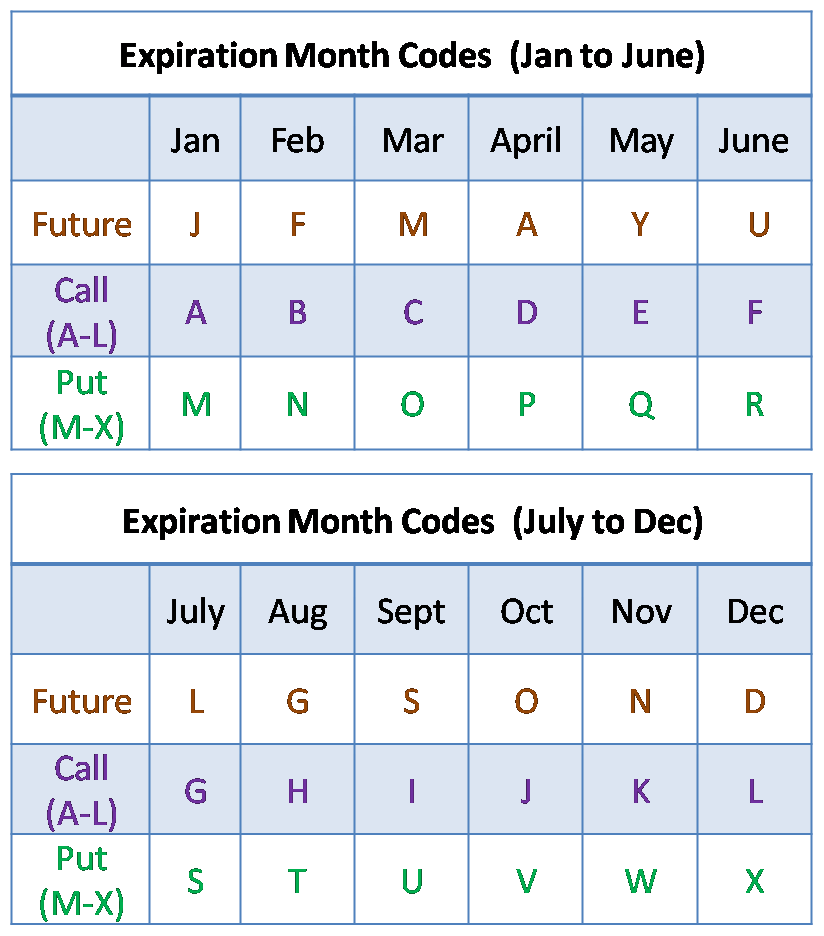# TrueData Velocity™ – Expiration Month Symbol Codes

These symbol codes can be used with Technical analysis applications (eg. Metastock) where the symbol length needs to be restricted to 14 characters.

All other TA apps, can also use the standard exchange symbology.

TrueData Velocity Symbol codeExample Symbols (Please refer the code tables):

• NIFTY 03/2015 (March 2015) Future = NIFTY+M+5 = NIFTYM5

Underlying (NIFTY) + M (March) + 5 (Exp. Year)

• NIFTY Put 12/2015 2900 = NIFTY+X+5+2900 = NIFTYX52900

Underlying (NIFTY) + X (Dec Put) + 5 (Exp Year 2015) + 2900 (Strike)

• NIFTY Call 12/2016  4300NIFTY+L+6+4300 = NIFTYL64300

Underlying (NIFTY) + L (Dec Call) + 6 (Exp Year 2016) + 4300 (Strike)